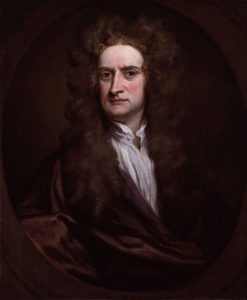# Isaac Newton

Sir Isaac Newton was an English mathematician, physicist, astronomer, theologian and author. His infamous scientific discoveries make him of the most influential scientists of all time.

Isaac Newton was in 1643 in England. When he attended Trinity College in Cambridge University in 1661, where he discovered the generalized binomial theorem and began to develop a mathematical theory that later became known as calculus. He also discovered Newton’s identities, Newton’s method, classified cubic plane curves, made substantial contributions to the theory of finite differences, and was the first to use fractional indices and to employ coordinate geometry to derive solutions to Diophantine equations. Later on, he worked on mechanics, publishing the three universal laws of motion in 1687, laying the foundation for classical mechanics. These contributed to many advances during the Industrial Revolution and were not improved for more than 200 years. He also recognized the deviation of the Sun from the center of gravity of the Solar System. Additionally, Newton found 72 out of 78 species of cubic curves and categorized them into 4 types. He also built the first practical reflecting telescope and he used his description of gravity to derive Kepler’s laws of planetary motion, account for tides, the trajectories of comets and the precession of the equinoxes.